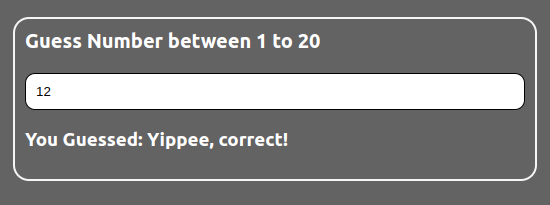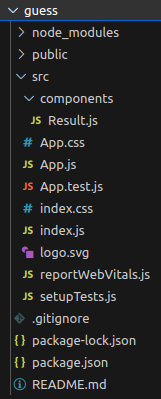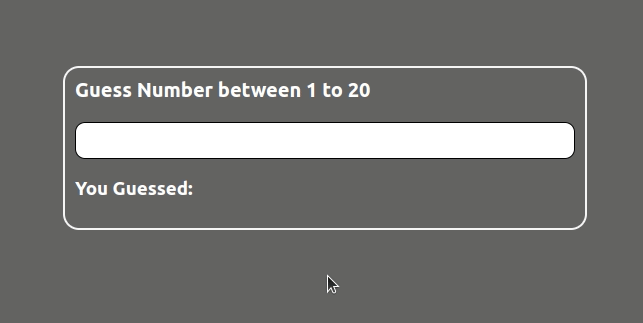Open In App
Related Articles

# Guess the number with React

In this article, we will create the guess the number game. In which the computer will select a random number between 1 and 20 and the player will get unlimited chances to guess the number. If the player makes an incorrect guess, the player will be notified whether the guess is is higher or lower than correct number until the correct guess is made.

To achieve this we will create two components App and Result. App components contain all the logic, it is stateful and the Result component only shows the appropriate message on the page according to the user’s guess. There is a default prop we set to the App component ‘secret’ that holds the required secret number and it is generated randomly.

Lets have a look at how the final application will look likePrerequisite:

Steps to create the projects:

Step 1: Initialize the project by using the following command

`npx create-react-app guess`

Step 2: Navigate to the folder using the command

`cd guess`

Step 3: Create a folder named components in src folder and add a new file in it named Result.js

After following the above steps the project structure will look like:

Project Structure:The dependencies in package.json will look like:

package.json

```"dependencies": {
"@testing-library/jest-dom": "^5.16.5",
"@testing-library/react": "^13.4.0",
"@testing-library/user-event": "^13.5.0",
"react": "^18.2.0",
"react-dom": "^18.2.0",
"react-scripts": "5.0.1",
"web-vitals": "^2.1.4"
}```

Example : Write the following code in different files.

• App.js: This files contains the logic and imports the required component
• App.css: This file contains the styling of the application
• Result.js: This file displays the result based on the input

## Javascript

 `// App.js` `import React, { Component } from ``'react'``import Result from ``'./components/Result'``import ``'./App.css'``class App extends Component {` `    ``static defaultProps = {``        ``secret: Math.floor(Math.random() * 20) + 1``    ``}` `    ``constructor(props) {``        ``super``(props)``        ``this``.state = { term: ``''` `}` `        ``this``.handleChange = ``this``.handleChange.bind(``this``)``    ``}` `    ``handleChange(event) {``        ``this``.setState({``            ``[event.target.name]: event.target.value``        ``})``    ``}` `    ``render() {``        ``return` `(``            ``
``                ``
``                    ````                ``
``                ``` `                ````            ``
``        ``)``    ``}``}``export ``default` `App`

## CSS

 `/* App.css */` `body {``    ``background-color``: ``rgb``(``100``, ``99``, ``99``);``    ``display``: flex;``    ``align-items: ``center``;``    ``justify-``content``: ``center``;``    ``padding``: ``15%``;``}` `.container {``    ``padding``: ``10px``;``    ``border``: ``2px` `solid` `whitesmoke;``    ``width``: ``500px``;``    ``border-radius: ``16px``;``    ``display``: flex;``    ``flex-``direction``: column;``    ``color``: ``rgb``(``255``, ``255``, ``255``);``}` `.head {``    ``font-weight``: ``600``;``    ``font-size``: ``20px``;``    ``margin-bottom``: ``20px``;``}` `input {``    ``padding``: ``10px``;``    ``border``: ``1px` `solid` `black``;``    ``border-radius: ``10px``;``}` `input:hover {``    ``background-color``: ``rgb``(``158``, ``156``, ``152``);``}` `input:focus {``    ``border``: ``0px``;``    ``background-color``: beige;``}`

## Javascript

 `// Result.js` `import React from ``'react'` `const Result = ({ term, secretNum }) => {``    ``let result;``    ``if` `(term) {``        ``if` `(secretNum > term) {``            ``result = ``'Lower'``        ``}``        ``else` `if` `(secretNum < term) {``            ``result = ``'Higher'``        ``}``        ``else` `if` `(secretNum == term) {``            ``result = ``'Yippee, correct!'``        ``}``        ``else` `{``            ``result = ``"Enter Valid Input"``        ``}``    ``}``    ``return` `

You Guessed: {result}

``}` `export ``default` `Result`

Steps to run the application:

Step 1: Type the following command in terminal

`npm start`

Step 2: Type the following link in you browser

`http://localhost:3000/`

Output: